# Variable-directions method

(diff) ← Older revision | Latest revision (diff) | Newer revision → (diff)

An iterative method (cf. Iteration methods) for solving systems of linear or non-linear equations arising in difference or projection-difference methods for the approximate solution of, for example, boundary value problems for partial differential equations of elliptic type.

Let, for example, there be two spatial variables and a sequence of square gridswith stepand nodes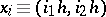, where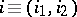is a vector with integer components. Let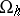be the set of nodes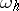at which one seeks a solution to a difference or projection-difference problem written in the form of the operator equation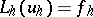in the Euclidean space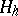. The latter can be identified with the space of functions given at the nodes of; the dimension ofcoincides with the number of pointsfrom.

Let(1)

be linear operators mappinginto. Among the operators (1) there are such for which the non-zero coefficientsin (1) correspond only to shift vectors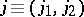having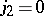. Such operators are called one-dimensional, acting with respect to, and they are denoted by; similarly, for shift vectors having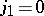, one defines the one-dimensional operators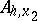acting on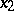.

The systems of equations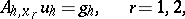split up into individual subsystems, each of which links only the values of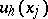at nodes lying on separate horizontal (for) or vertical (for) lines in the grid. The method is characterized by the use of intertwining operators for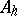of the form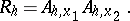The solution to the system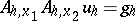(2)

then amounts to the successive solution of the two systems(3)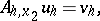(4)

in which one first solves the separate subsystems on the horizontal lines in the grid (in the case (3)) and then varies the directions and solves the subsystems on the vertical lines (in the case (4)). Usually, the operators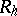are taken such that only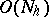arithmetic operations are involved in solving (3) and (4), and consequently also (2). Therefore, each iteration in the method, of the form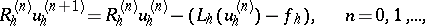(5)

requiresarithmetic operations, where the superscriptcorresponds to the number of the iteration.

The most effective results are obtained for the commutative case, whereis a self-adjoint positive-definite operator, while the operators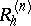are self-adjoint and commute with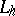. In that case, for anythe error in the initial approximation can be reduced in norm by a factor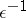in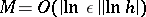iterations. The commutative case will be encountered only for boundary value problems in which the variables can be separated, and therefore, the region should be a rectangle. The most common case of the method (5) for the equationis the methodwhere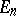is the identity operator.

One also uses approaches based on different variational principles, supplementing the approach in which the iteration parameters are chosen to minimize the norm of the operator for passing from the zero-th iteration to an iteration of a given index.

The method is frequently used as an internal iterative process in two-step iteration methods based on operators that are equivalent in spectrum and suitable for use with variable coefficients and non-linear problems.

How to Cite This Entry:
Variable-directions method. Encyclopedia of Mathematics. URL: http://encyclopediaofmath.org/index.php?title=Variable-directions_method&oldid=11482
This article was adapted from an original article by E.G. D'yakonov (originator), which appeared in Encyclopedia of Mathematics - ISBN 1402006098. See original article